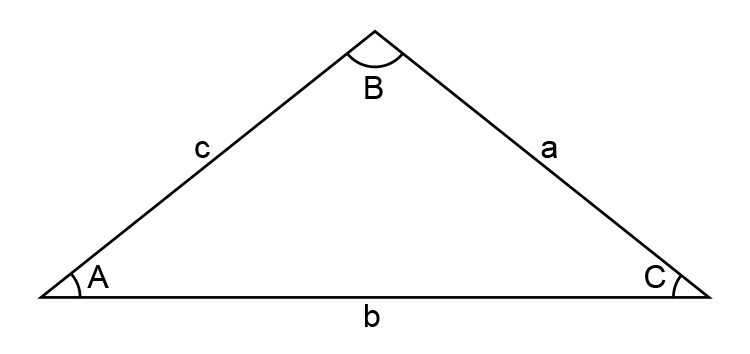# Cosine rule

Cosine rule – We can use the cosine formula to find the length of a side or the size of an angle.a^2=b^2+c^2-2bc\cosA

The following mnemonic (picture memory aid) is what we recommend to remember this formula.Zero

That’s correct. We recommend nothing. We recommend that you do not remember this formula. You don’t need it.

See examples NOT using cosine rule.